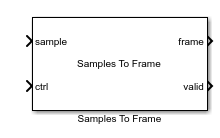# Samples To Frame

Convert sample stream to frame-based data

• Library:
• Wireless HDL Toolbox / I/O Interfaces

•## Description

The Samples To Frame block reconstructs frame-based data from a stream of samples and its corresponding control signals. It removes any idle or nonvalid samples from the data. See Streaming Sample Interface for details of the streaming format.

Use this block to process output from a subsystem targeted for HDL code generation. This block does not support HDL code generation.

## Ports

### Input

expand all

Stream of samples, specified as a scalar or vector. Vector input values represent a single sample, such as turbo-encoded samples represented by one systematic value and two parity values. The stream can include idle cycles between samples and between frames. Idle samples are discarded. `double` and `single` are supported for simulation but not for HDL code generation.

Data Types: `single` | `double` | `int8` | `int16` | `int32` | `uint8` | `uint16` | `uint32` | `Boolean` | `fixed point`

Control signals accompanying the sample stream, specified as a `samplecontrol` bus. The bus includes the `start`, `end`, and `valid` control signals, which indicate the boundaries of the frame and the validity of the samples.

• `start` — Indicates the start of the input frame

• `end` — Indicates the end of the input frame

• `valid` — Indicates that the data on the input data port is valid

For more details, see Sample Control Bus.

Data Types: `bus`

### Output

expand all

Frame of output samples, returned as a column vector. Each frame is the same size. If the input frame is smaller than Output size, the block pads the frame with zeroes. If the output frame is larger than the Output size, the block forms the frame by using the first Output size samples. You can optionally output the number of valid samples in each frame on the len port.

Validity of output frame, returned as a `Boolean` scalar. This port returns `1` (`true`) when the values on the frame and len (optional) ports, are valid.

Data Types: `Boolean`

Number of valid samples in output frame, returned as an integer. The input sample stream can have frames of different sizes. The block returns a constant size vector on the frame port, padded with zeroes when the input frame is smaller than Output size. The len port indicates how many valid samples are in the output frame. If the output frame is larger than the Output size, the block forms the frame by using the first Output size samples.

Data Types: `double`

## Parameters

expand all

Number of values representing each sample, specified as a positive integer scalar. The block accepts a vector of Input size values. Each vector has one corresponding set of control signals. For example, you can use this parameter for turbo-encoded samples. In the LTE standard, the turbo code rate is 1/3, so each sample is represented by one systematic value and two parity values: S_n, P1_n, and P2_n. In this case, set Input size to `3`.

Number of input cycles to buffer before attempting to form an output frame, specified as an integer. The block simulates faster when this parameter is larger. However, the block returns at most one frame from each search window. If more than one frame fits in this window, the block returns the first one it finds and drops the later frames. The default setting, 1 cycle, never drops frames, but results in slower simulation. Therefore, it is a best practice to set this parameter to the minimum number of cycles per frame, including idle cycles.

For example, calculate the valid cycles and idle cycles representing each frame. Each cycle may include more than one sample, depending on your Input size (samplesize) setting.

```% Exact setting: includes idle cycles totalframesize = ((framesamples/samplesize)*... (idlecyclesbetweensamples+1))+idlecyclesbetweenframes;```
If the frame and sample spacing is variable or unknown, then a conservative compromise is to set the Frame search window to the minimum number of valid cycles per frame. For instance, for a turbo encoder block, the output frame size depends on the coding rate, 1/R, and tail bits specified by the LTE standard. The output data has R samples per cycle. This calculation does not include any idle cycles between samples or between frames.
```% Conservative setting: number of valid output cycles, without idles encoderrate = 3; numtailbits = 12; framesize = (framesamples+numtailbits)/encoderrate;```

Maximum number of samples per frame, specified as an integer. The input sample stream can have frames of different sizes. The block returns a constant size vector, padded with zeroes if the frame is smaller than Output size. If the block receives a frame larger than Output size, it truncates the frame.

Order of output samples relative to input order, when more than one value represents each sample. For example, 1/3 turbo-encoded samples are represented by `[S_1 P1_1 P2_1]` and ```[S_2 P1_2 P2_2]```. The default output order is ```[S_1 P1_1 P2_1 S_2 P1_2 P2_2]```. To reorder the samples so that systematic and parity values are grouped together, select Compose output from interleaved input samples. The output order is then `[S_1 S_2 P1_1 P1_2 P2_1 P2_2]`.

Enable frame length output port. Select this option to return the number of valid samples in each output frame. The length is returned on the len port and is qualified by the valid signal. Use this option when the sample stream has variable size frames or when a downstream block requires the frame size as input, such as LTE Turbo Decoder.

## Version History

Introduced in R2017b